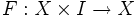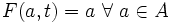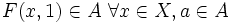# Deformation retraction

This article defines a property of a homotopy from a topological space to itself

## Definition

Let$X$ be a topological space and$A$ a subspace. A deformation retraction from$X$ to$A$ is a homomotopy$F: X \times I \to X$ such that:

•$F(x,0) = x \ \forall \ x \in X$
•$F(a,t) = a \ \forall \ a \in A$
•$F(x,1) \in A \ \forall x \in X, a \in A$

If a deformation retraction exists from$X$ to$A$, we say that$A$ is a deformation retract of$X$.